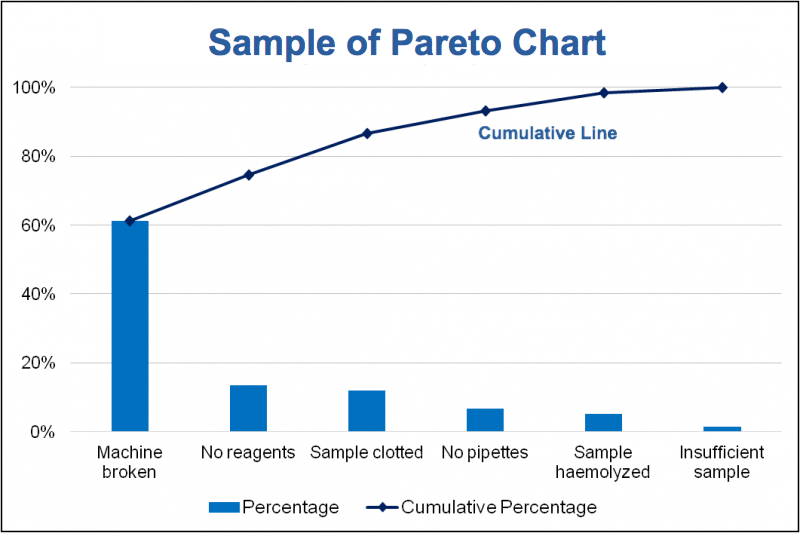# Introduction of 7 QC tools for process Improvement with Examples

Updated: Aug 29, 2020

Introduction of 7 QC tools: 7 QC tools comprised of simple graphical and statistical techniques were helpful in solving Critical to Quality (CTQ's) related issues. These Quality tools were often referred to as 'Seven QC tools of Quality'.Introduction of 7 QC tools for process Improvement with Examples

7 QC tools are:

1. Cause & Effect diagram:

Cause & Effect diagram is also known as 'Ishikawa diagram' or 'Fishbone diagram'. This tool introduced by Kaoru Ishikawa. The Cause and Effect diagram helps us to identify the various causes (Factor) leading to an effect (Problem).

The main purpose of the use of this diagram is: To understand the root cause behind the problem statement. To identify the source of variation the causes are usually grouped into these categories (According to the team understanding):

You Can Use 6 M for Fishbone diagram: 1. Man 2. Machine 3. Material 4. Method 5. Measurement and 6. Mother Nature or environmental.

You can also use 5 P: 1. People 2. Process 3. Product 4. Policy 5. ProcedureIntroduction Cause & Effect diagram: Example

2. Histogram:

The Histogram was introduced by Karl Pearson. The main purpose of the use is plotting the shapes of distribution and comparing it with the specification.

How to use Histogram: Prepare separately, the stratified histogram for each of the 4M's and examine the relationship between the shapes of the distributions and the specifications. Use at least 30 values, preferably 100 values for mass manufacturers. more in data, more in conclusion.Introduction of Histogram and examples

3. Pareto Chart:

Pareto Chart was introduced by Vilfredo Pareto. The Pareto chart is revolved around the 80-20 rules, Which underline that in any process 80% of the problems is just caused by 20% of few major factor which is referred as vital few, whereas remaining 20% of the problem is caused by 80% of many minor factors which is referred as trivial many.

The purpose to use the Pareto chart to identify and priorities the problem, Analysing symptoms, and proving the effectiveness of the remedy.Introduction of Pareto Chart and examples

4. Check sheet:

The purpose of the use of the checklist is to list down the Important checkpoints or events in a tabular/ Metrics format and keep on updating or marking the status on their occurrence which helps in understanding the progress, defect pattern and even cause for the defect.Introduction of Check sheet and example

5. Flow Chart:

The flow chart is a type of diagram which represents a workflow or process through the boxes.

Purpose of flow Chart:

A visual illustration of the sequence of operations required to complete a task.

1. Schematic drawing of the process to measure the defects and improve.

2. Identify the potential weakness in the process is made visual.

3. Picture of the process as it should be.

What are the benefits of the flow chart

1. Identifying the process difficulty very easily

2. Understand the process

3. Identify the duplicate effort and non-value added steps

4. Clarify working relationships between people and organizationWhat is Flow Chart with example. Benefits of the Flow Chart

6. Scatter Diagram:

A Scatter diagram is also called a scatter plot. Both are basically a statistical tool that depicts the dependent variable on Y-Axis and the independent variable on the X-Axis plot as a dot on their common intersection points.

Purpose of the scatter diagram: To identify the correlation between two variables (X and Y). Joining these dots can highlight any existing relationship among these variables or an equation in format Y=F(X)+C. C is an arbitrary constant.

The relationship can be linear, curvilinear, Exponential, logarithmic, quadratic, polynomial. Stronger the correlation, Stronger the relationship will hold true.Introduction of Scatter Diagram

7. Control Chart:

The control chart is also known as the Shewhart chart. The control chart was introduced by Walter A. Shewhart. The Control chart is a basically statistical chart that helps in determining if any industrial process or products are within the control limit and capable to deliver or meet the customer's defined specification limit.

Why we use a control chart?

1. Predict process out of control or out of specification limits.

2. Distinguish between specific, identifiable causes of variations.

3. Can be used for SPC (Statistical Process Control).Introduction of Control Chart

81 views0 comments

### Recent Posts

See All

#### What is Pareto? History of Pareto Chart & How to Make Pareto Chart In Excel.All Rights reserve for Quality Duniya 2020

Thanks for submitting!